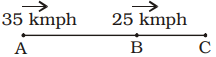## Speed, Time and Distance

#### Speed, Time and Distance

1. A train ‘B’ speeding with 100 kmph crosses another train C, running in the same direction, in 2 minutes. If the length of the train B and C be 150 metre and 250 metre respectively, what is the speed of the train C (in kmph)?
1. 75
2. 88
3. 95
4. 110

1. Let the speed of train C be x kmph.
∴  Relative speed of B
= (100 – x ) kmph.

 ∴  Time taken in crossing = Length of both trains Relative speed

 ⇒  (2/60) =150 + 2501000 100 − x

 ⇒ 1 = 2 30 5(100 − x)

 ⇒ 1 = 2 6 100 − x

⇒  100 – x = 12
⇒  x = 100 – 12 = 88 kmph.

##### Correct Option: B

Let the speed of train C be x kmph.
∴  Relative speed of B
= (100 – x ) kmph.

 ∴  Time taken in crossing = Length of both trains Relative speed

 ⇒  (2/60) =150 + 2501000 100 − x

 ⇒ 1 = 2 30 5(100 − x)

 ⇒ 1 = 2 6 100 − x

⇒  100 – x = 12
⇒  x = 100 – 12 = 88 kmph.

1. The distance between two places A and B is 60 km. Two cars start at the same time from A and B, travelling at the speeds of 35 km/h and 25 km/h, respectively. If the cars run in the same direction, then they will meet after ( in hours)
1. 6.5
2. 6.2
3. 6
4. 6.52

1.Let both cars meet at C after t hours.
∴  Distance covered by car A
= AC = 35t km
Distance covered by car B
= BC = 25t km
∴  AC – BC = AB = 60 km.
⇒  35t – 25t = 60
⇒  10t = 60

 ⇒  t = 60 = 6 hours 10

##### Correct Option: CLet both cars meet at C after t hours.
∴  Distance covered by car A
= AC = 35t km
Distance covered by car B
= BC = 25t km
∴  AC – BC = AB = 60 km.
⇒  35t – 25t = 60
⇒  10t = 60

 ⇒  t = 60 = 6 hours 10

1. Two trains start from stations A and B and travel towards each other at speeds of 50 kmph and 60 kmph respectively. At the time of their meeting, the second train has travelled 120 km more than the first. The distance between A and B is
1. 1200 km
2. 1440 km
3. 1320 km
4. 990 km

1. Let both trains meet after t hours.
∴   Distance = speed × time
∴  60t – 50t = 120
⇒  10t = 120 ⇒ t = 12 hours
∴  Required distance
= 60t + 50t
= 110t = 110 × 12
= 1320 km

##### Correct Option: C

Let both trains meet after t hours.
∴   Distance = speed × time
∴  60t – 50t = 120
⇒  10t = 120 ⇒ t = 12 hours
∴  Required distance
= 60t + 50t
= 110t = 110 × 12
= 1320 km

1. Two trains of equal length are running on parallel lines in the same direction at the rate of 46 km/hr and 36 km/hr. The faster train passes the slower train in 36 seconds. The length of each train is
1. 50 m
2. 72 m
3. 80 m
4. 82 m

1. Length of each train = x metre
Relative speed = 46 – 36 = 10 kmph

 =10 × 5m/sec 18

 = 25 m/sec 9

 ∴  Time taken in crossing = Length of both trains Relative speed

 ⇒ 36 = 2x 25 9

 ⇒  2x = 36 × 25 = 100 9

 ⇒  x = 100 = 50 metre 2

##### Correct Option: A

Length of each train = x metre
Relative speed = 46 – 36 = 10 kmph

 =10 × 5m/sec 18

 = 25 m/sec 9

 ∴  Time taken in crossing = Length of both trains Relative speed

 ⇒ 36 = 2x 25 9

 ⇒  2x = 36 × 25 = 100 9

 ⇒  x = 100 = 50 metre 2

1. A thief steals a car at 1.30 p.m. and drives it off at 40 km/hr. The theft is discovered at 2 p.m. and the owner sets off in another car at 50 km/hr. He will overtake the thief at
1. 5 p.m.
2. 4 p.m.
3. 4.30 p.m.
4. 6 p.m.

1. Distance covered by the thief in half an hour

 = 1 × 40 = 20 km 2

Relative speed of car owner
= 50 – 40 = 10 km
 ∴   Required time = Difference of distance Relative speed

 = 20 = 2 hours 10

i.e. at 4 p.m.

##### Correct Option: B

Distance covered by the thief in half an hour

 = 1 × 40 = 20 km 2

Relative speed of car owner
= 50 – 40 = 10 km
 ∴   Required time = Difference of distance Relative speed

 = 20 = 2 hours 10

i.e. at 4 p.m.